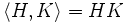# Permutable implies modular

This article gives the statement and possibly, proof, of an implication relation between two subgroup properties. That is, it states that every subgroup satisfying the first subgroup property (i.e., permutable subgroup) must also satisfy the second subgroup property (i.e., modular subgroup)
View all subgroup property implications | View all subgroup property non-implications

## Statement

Any permutable subgroup of a group is modular.

## Definitions used

### Permutable subgroup

Further information: Permutable subgroup

A subgroup$H$ of a group$G$ is termed permutable if$HK = KH = \langle H, K \rangle$ for every subgroup$K \le G$.

### Modular subgroup

Further information: Modular subgroup

## Facts used

1. Modular property of groups: This states that if$H,K,L$ are subgroups of$G$ such that$H \le L$, then:$H(K \cap L) = HK \cap L$.

## Proof

Given: A subgroup$H$ of a group$G$ such that$HK = KH = \langle H, K \rangle$ for all subgroups$K \le G$.

To prove: For any subgroups$K, L$ of$G$ such that$H \le L$, we have:$\langle H, K \cap L \rangle = \langle H, K \rangle \cap L$.

Proof: Since$H$ is permutable, we have:$\langle H, K \cap L \rangle = H(K \cap L)$.

and:$\langle H, K \rangle = HK$, so$\langle H, K \rangle \cap L = HK \cap L$.

Applying fact (1) now yields the result.